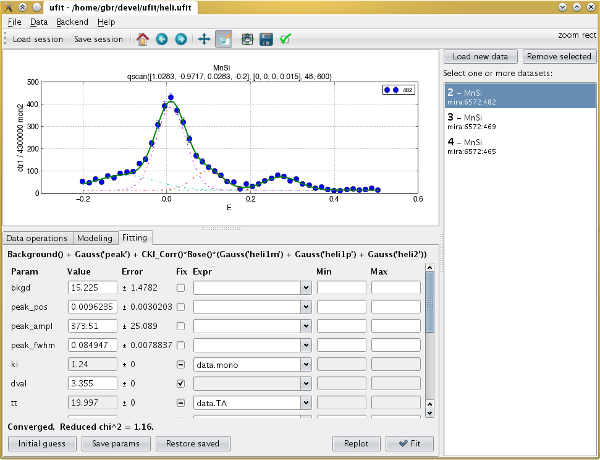# ufit overview¶

ufit is a universal neutron scattering data fitting package for use both in noninteractive scripts, and with a graphical user interface.

It handles complex fitting functions by combining them from pre-defined Pythonic “model” objects and handling parameter constraints and interdependencies well.

Fitting is done by one of several backends:

• lmfit‘s Levenberg-Marquardt least-squares that allows parameter constraints
• Minuit’s “migrad” algorithm (via pyminuit 1 or 2)
• Basic Levenberg-Marquardt least-squares from scipy.optimize

Results can be easily plotted and further processed. Plotting is handled by matplotlib.

The GUI uses PyQt 4.

Contents:

Sample script usage:

```# import all ufit API plus pylab
from ufit.lab import *

# set a template, so that data can be referenced by number only
set_datatemplate('path/to/data%04d.dat')
# read one dataset from a file, with given X and Y columns
data = read_data(104, 'A3', 'CNTS')

# create a model of a simple Gaussian peak with given initial guess
model = Background() + Gauss('peak', pos=97.5, ampl=100, fwhm=0.5)

# fit the model, then print and plot the result
result = model.fit(data)
result.printout()
result.plot()
show()
```

GUI screenshot: